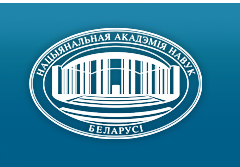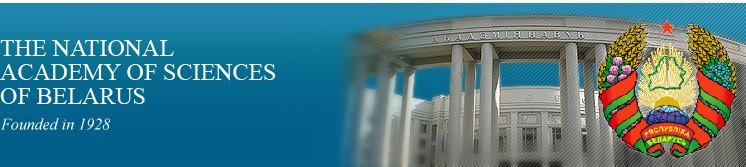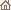Home News About Academy Academy Activities Academy Membership Organizations Publications Conferences Contacts Site Map

# Proc. of the Natl. Academy of Sciences of Belarus, Ser. Phys.-Math. Sci., 2012, No.1/ Publications / Scientific Journals

### Number 1, 2012## CONTENTS

### MATHEMATICS

Gorokhovik V. V., Naidenko V. G.
Epi-Lipschitzian property of directed half-spaces of restricted-orientation convexity. pp. 4-7.

Summary: We prove that each closed directed halfspace of restricted-orientation convexty on Rn is an epi-Lipschitzian set provided the restricted-orientation convexty is spatial. Using this result we give a positive solution of Fink-Wood conjecture that the boundary of any directed half-space of restricted-orientation convexity holding a point-intersection property is path connected.

Egorov A. D., Ulasik A. F.
Approximate formulas of third order exactness for evaluation of the mathematical expectation of functional from the solution to the stochastic equation. pp. 8-12.

Summary: Approximate formulas for evaluation of the mathematical expectation of functional from the solution to Ito’s inhomogeneous linear equation are constructed. The formulas are exact for third degree functional polynomials of the solution.

Hudyakov A. P.
Explicit formulas of errors for one case of Hermite interpolation. pp. 13-21.

Summary: The article contains explicit formulas of errors and their estimates for polynomials of Hermite–Birkhoff type with respect to trigonometric, rational and exponential functions.

Rasolko G. A.
Method for exact solution of the first-kind singular integral equation with the Cauchy kernel and a special right hand-side. pp. 22-26.

Summary:Shiryaev V. M.
Construction of finite quasi-commutative m-rings. pp. 27-33.

Summary: The article deals with quasi-commutative m-rings, i. e. such m-rings (K, +,×, o), in which aob and boa generate the same subring of the ring (K,+,×). Being finite and possessing the identity, such a m-ring K is an extention of a nilpotent m-ring by a boolean m-ring in which the operation of multiplication coincides with that of superposition.

Vorob’ev N. N., Mekhovich A. P.
One class of directly reducible generated saturated formations. pp. 34-38.

Summary:Shlyk V. A.
Approximate representation of integer numbers by sums of integer algebraic numbers. pp. 39-41.

Summary: We give a bound on the number of times a sum of two integer algebraic numbers is in a given neibourhood of a fixed integer number.

Emelichev V. A., Korotkov V. V.
Stability of the vector Boolean portfolio optimization problem with Savage’s regularized risk criteria. pp. 42-47.

Summary: We consider a vector Boolean portfolio optimization problem with Savage’s regularized minimax risk criteria. We obtained lower and upper attainable bounds for the stability radius of the problem in the case of the linear metric l1 in the portfolios space, and Chebyshev’s metric lµ in the risk and market conditions space.

Vasilevsky K. V., Lomovtsev F. E.
Boundary-value problem for a complete third-order operator differential equation with variable domains of unbounded operators. pp. 48-54.

Summary: The existence and uniqueness theorems of weak solutions of the boundary-value problem for a complete third-order operator-differential equation with variable domains of unbounded operator coefficients are proved.

Vardanian R. S.
Characteristics of the radiation field in the semi-infinite stochastic media. pp. 55-61.

Summary: The problem of radiation transfer in the semi-infinite medium is considered under the assumption that the probability of quantum survival for an elementary act of scattering is expressed by statistically homogeneous. Gaussian field with an exponential correlation function. The corresponding Dyson equation is solved by means of the method of nonlinear equations of integral operator factorization. The first moments of the source function and of the intensity as well as the average number of quantum scatterings and the reflection coefficient are calculated.

### PHYSICS

Chernichenko Yu. D.
Relativistic quasi-potential equation with a linear potential in the case of arbitrary masses. pp. 62-68.

Summary: A solution of the integral equation with a linear quasi-potential for S-wave is obtained. Consideration is conducted within the framework of the relativistic quasipotential approach in quantum field theory formulated in the relativistic configurational representation in the case of two particles with arbitrary masses.

Ovsiyuk E. M., Tokarevskaya N. G.
Nonrelativistic description of particle with spin 1 in the external Coulomb field. pp. 69-75.

Summary: A quantum-mechanical system – a particle with spin 1 in the external Coulomb field is investigated on the basis of the Duffin – Kemmer matrix aproach involving the tetrad formalism. With the use of the operator of spatial inversion, the system of 10 radial equations is splited into two subsystems: 4 equations for states with a parity P=(-1)j+1, and 6 equations for states with a parity P=(-1)j. In these radial systems, the transition to the nonrelativistic limit is performed, and equations are then solved in terms of hypergeometric functions. The exact nonrelativistic energy spectrum for a vector particle is found in explicit form.

Andreev V. V., Pankov A. A.
Investigation of Z’-boson effects at CLIC with polarization. pp. 76-82.

Summary: The method to perform a model independent analysis of Z?-boson effects in the process e+ + e- ® W+ + W- was proposed by means of generic parameters x+1 and x-1, which describe the class of models with an extended gauge sector. Model independent constraints on the Z?-boson parameters were obtained by the proposed method. The role of polarized beams to improve the sensitivity to Z?-boson parameters of the process e+ + e- ® W+ + W-was studied.

Shulyakovsky R. G.
Tunneling processes in scalar field models. pp. 83-87.

Summary: Tunneling processes in scalar field models in limited space regions, which can be described by instantons, are considered. For two-dimensional models exact instanton solutions are given. Corresponding soliton solutions for three-dimensional models are also given. The possibility to take into account tunneling processes as external classical fields in the Euclidean space for scalar fields (in infinite space) is shown. Corresponding correction to Green’s fermion function is calculated for the model external field.

Andreev V. V.
Calculation of the equation kernel of the relativistic two-fermion system. pp. 88-95.

Summary: The kernel of the radial equation for the relativistic two-fermion system with arbitrary angular momentum was obtained. The procedure is based on the exact calculation of fermion elastic scattering amplitude being a part of the interaction potential definition.

Ryabushko A. P., Nemanova I. T., Zhur T. A.
Metric of the Riemann space-time due to the gravitating center surrounded by a nonhomogeneous medium. pp. 96-100.

Summary: The post-Newtonian approximation of the Einstein equations has been used to obtain and discuss the metric of the Riemann space-time, which appears around the gravitating center surrounded by a nonhomogeneous medium and is based on a polygonal three-element spherically symmetric model.

Tochitskii T. A., Trukhanov A. V., Dmitrieva A. E., Nemtsevich L. V.
The structure and magnetoresistive properties of nanocrystalline nickel films. pp. 101-105.

Summary: The formation of the structure and electrical resistance of nanocrystalline nickel films are investigated. The mechanism of a magnetic field and the texture influence on the film electrical resistance is presented.

Sheleg A. U., Hurtavy V. G., Mustafaeva S. N., Kerimova E. M.
Electrical conductivity and dielectric properties of Tl(InSe2)1–x(GaTe2)x single crystals. pp. 106-109.

Summary: The temperature dependence of conductivity and permittivity of solid solutions Tl(InSe2)1–x(GaTe2)x crystals in the temperature range 80–300 K are studied. It is established that the conductivity of the crystals increases with temperature and measuring field frequency, and the dielectric constant decreases with increasing frequency and concentration x. A pronounced dispersion of the permittivity, especially at high temperatures is found. It is shown that the anomalies are observed on the d= f(T) and x= f(T) curves in the form of peaks due to the presence of phase transitions in these crystals. It is established that the temperature of phase transitions in Tl(InSe2)1–x(GaTe2)x solid solutions with increasing concentration x smoothly decreases.

### INFORMATICS

Likhoded N. A., Sobolevsky P. I.
Tiling of iterative domains of algorithms defined by the intersection of parallelepipeds. pp. 110-116.

Summary: Parameterized tiling of nested loops and a problem of obtaining ranges of parameters of global and local loops are considered. A representation of the parameter ranges allowing us to avoid redundant computations for the case of the iterative domains presented by the intersection of two parallelepipeds is obtained.

Demidenko V. M.
Extension of Monge’s conditions to a class of functionals defined on the symmetric group. Part II. pp. 117-123.

Summary: Two generalizations of Monge’s classical conditions for a class of linear permutable functionals are proposed. These generalizations guarantee a sizeable decrease of a set of feasible solutions for the corresponding optimization problems and the achievement of a global optimum of these functionals on the identical permutation./ Publications / Scientific Journals / Back to Top of Page

Designed and maintained by Dr. Nikolai N. Kostyukovich. Last updated: March 6, 2013
Created with assistance of Mrs. Lyudmila V. Smolentseva
Copyright © 2012-2013 The National Academy of Sciences of Belarus
Copyright © 2012 The Belaruskaya Navuka Publishing House